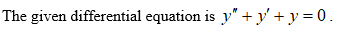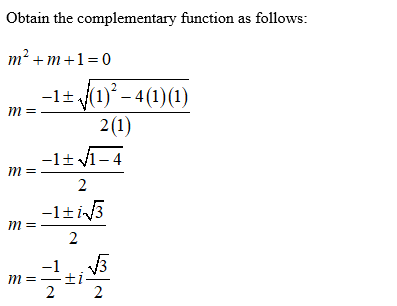# Solve the following differential equation: y′′+y′+y=0. The answer should be in the form y(x)= C1(...)+C2(...)

Question
1 views

Solve the following differential equation: y′′+y′+y=0. The answer should be in the form y(x)= C1(...)+C2(...)

check_circle

Step 1Step 2...

### Want to see the full answer?

See Solution

#### Want to see this answer and more?

Solutions are written by subject experts who are available 24/7. Questions are typically answered within 1 hour.*

See Solution
*Response times may vary by subject and question.
Tagged in

### Math